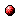DOC HOME SITE MAP MAN PAGES GNU INFO SEARCH

# (gmp.info.gz) Miscellaneous Float FunctionsInfo Catalog(gmp.info.gz) I/O of Floats(gmp.info.gz) Floating-point Functions
```
Miscellaneous Functions
=======================

- Function: void mpf_ceil (mpf_t ROP, mpf_t OP)
- Function: void mpf_floor (mpf_t ROP, mpf_t OP)
- Function: void mpf_trunc (mpf_t ROP, mpf_t OP)
Set ROP to OP rounded to an integer.  `mpf_ceil' rounds to the
next higher integer, `mpf_floor' to the next lower, and `mpf_trunc'
to the integer towards zero.

- Function: int mpf_integer_p (mpf_t OP)
Return non-zero if OP is an integer.

- Function: int mpf_fits_ulong_p (mpf_t OP)
- Function: int mpf_fits_slong_p (mpf_t OP)
- Function: int mpf_fits_uint_p (mpf_t OP)
- Function: int mpf_fits_sint_p (mpf_t OP)
- Function: int mpf_fits_ushort_p (mpf_t OP)
- Function: int mpf_fits_sshort_p (mpf_t OP)
Return non-zero if OP would fit in the respective C data type, when
truncated to an integer.

- Function: void mpf_urandomb (mpf_t ROP, gmp_randstate_t STATE,
unsigned long int NBITS)
Generate a uniformly distributed random float in ROP, such that 0
<= ROP < 1, with NBITS significant bits in the mantissa.

The variable STATE must be initialized by calling one of the
`gmp_randinit' functions ( Random State Initialization)
before invoking this function.

- Function: void mpf_random2 (mpf_t ROP, mp_size_t MAX_SIZE, mp_exp_t
EXP)
Generate a random float of at most MAX_SIZE limbs, with long
strings of zeros and ones in the binary representation.  The
exponent of the number is in the interval -EXP to EXP (in limbs).
This function is useful for testing functions and algorithms,
since these kind of random numbers have proven to be more likely
to trigger corner-case bugs.  Negative random numbers are
generated when MAX_SIZE is negative.

```Info Catalog(gmp.info.gz) I/O of Floats(gmp.info.gz) Floating-point Functions
automatically generated byinfo2html